## 算法有点甜之主席树

2018-03-01 4,559 次浏览

• 线段树（权值线段树）
• 离散化

• 区间/树上路径第 K 大
• 区间不同数的个数

### 主席树的建树过程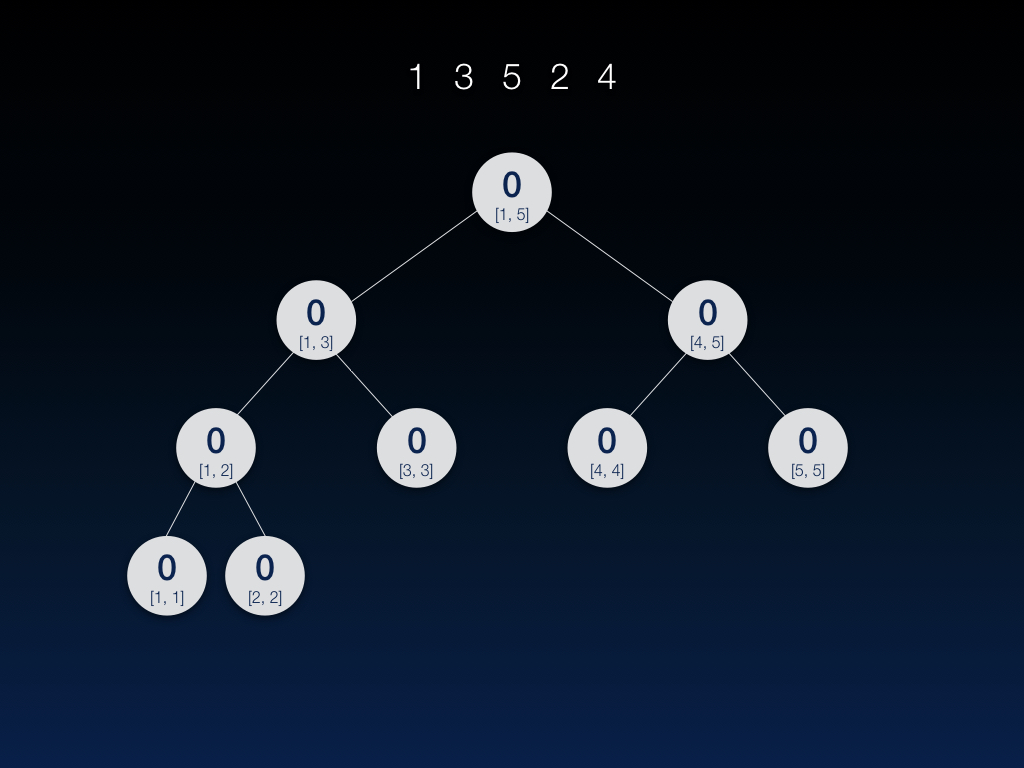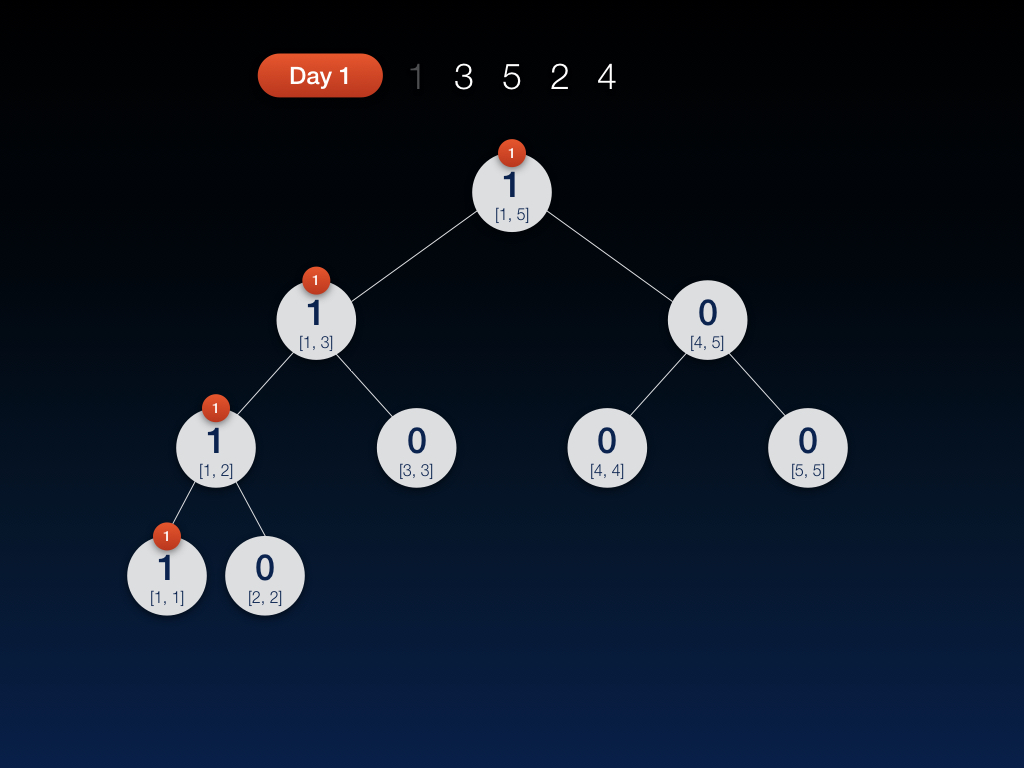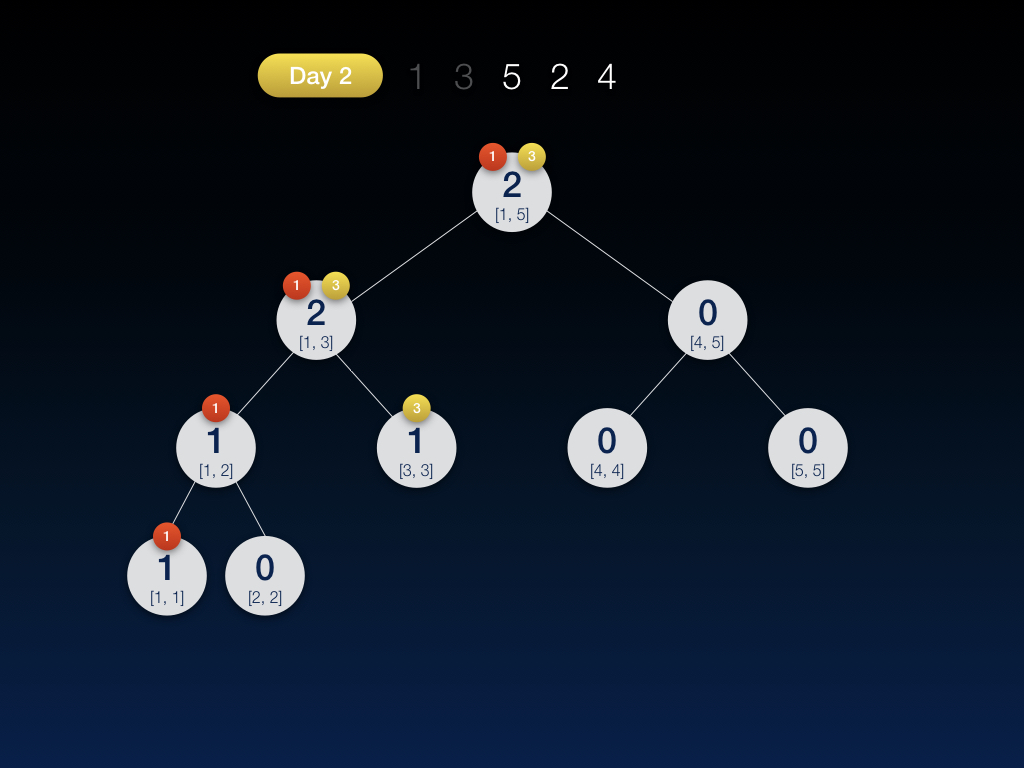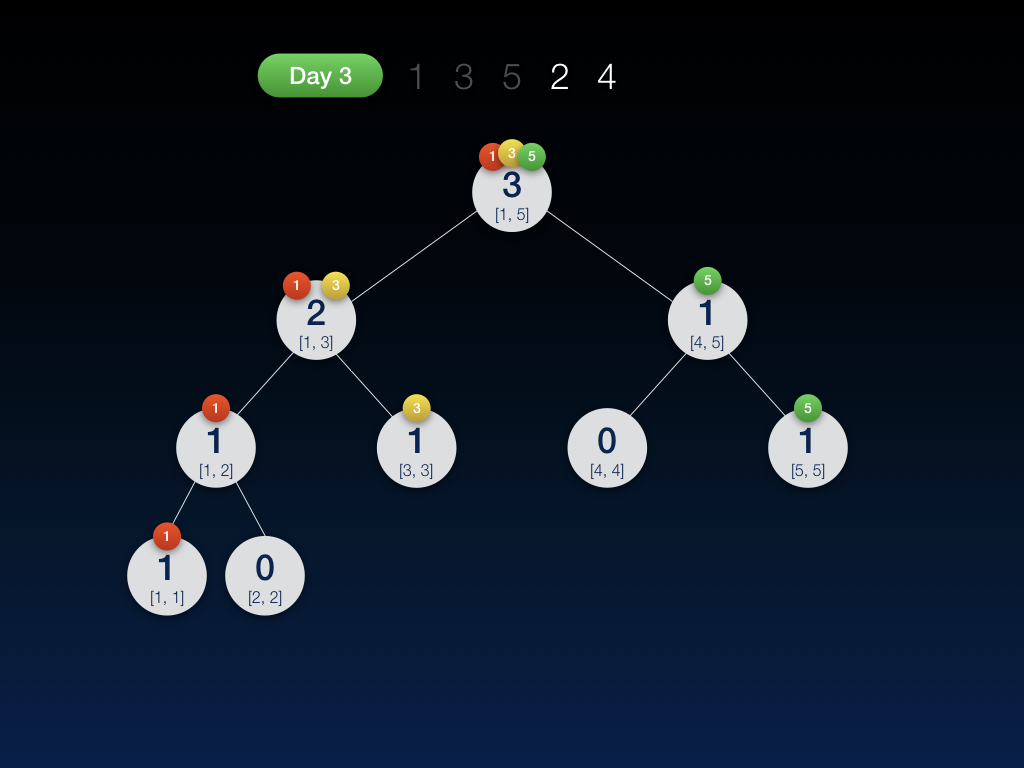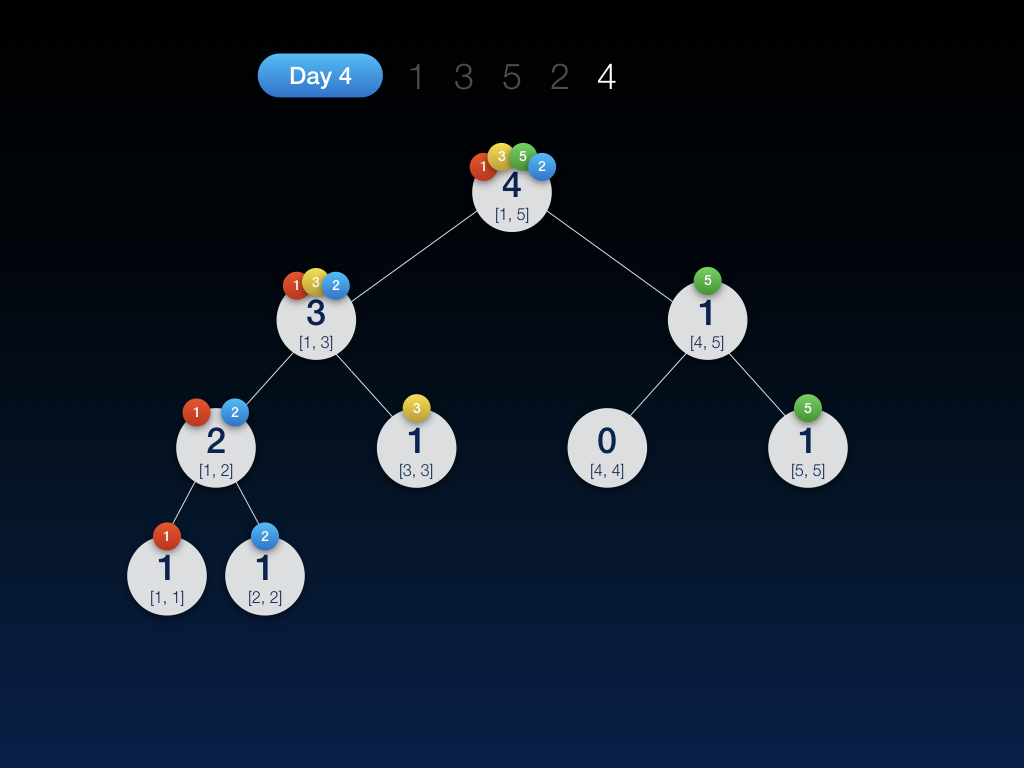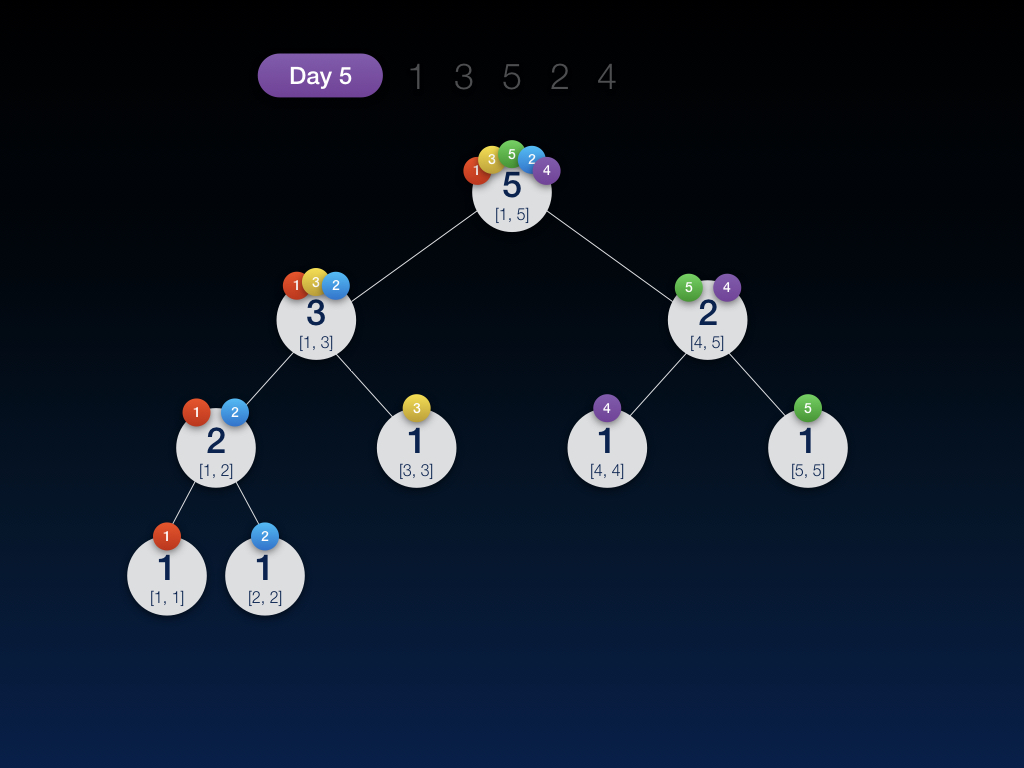OK！插入完毕，接下来我们来瞅一下，现在手里有这 5 天的总计 5 张线段树快照：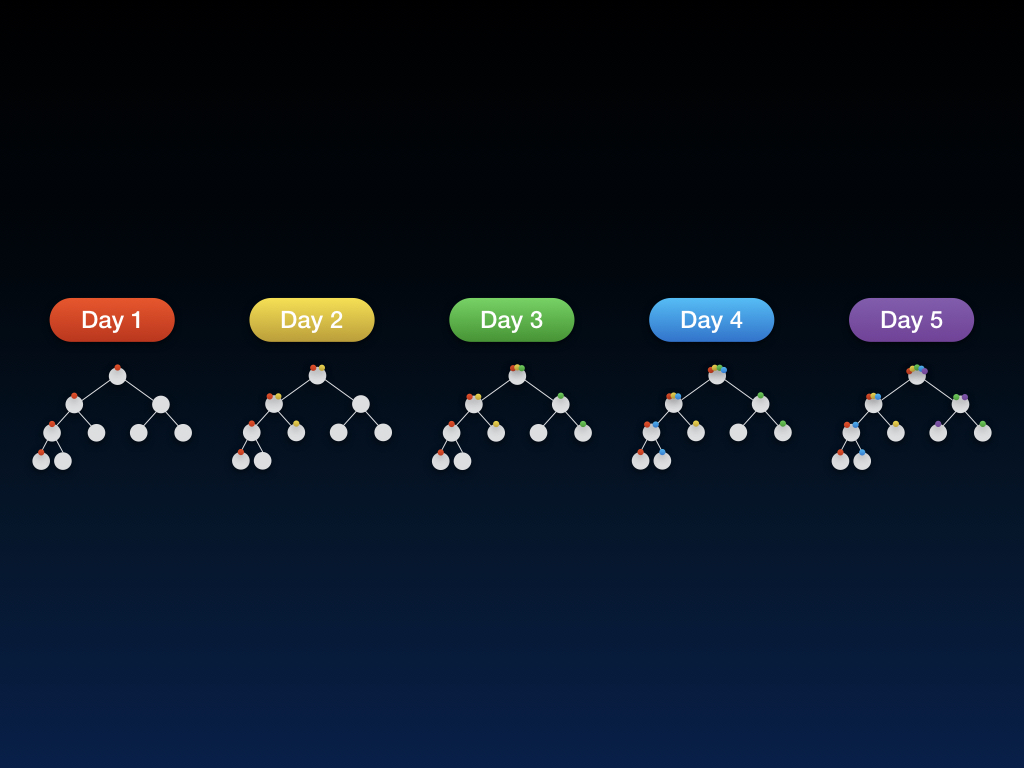### 主席树的重要性质：线段树做差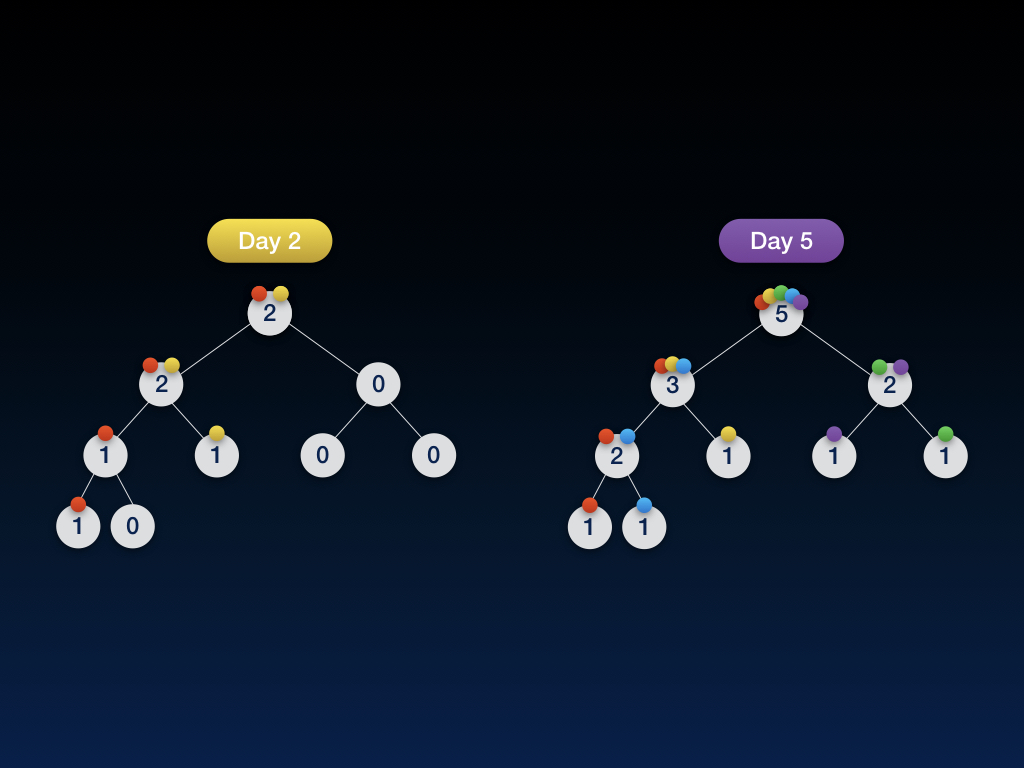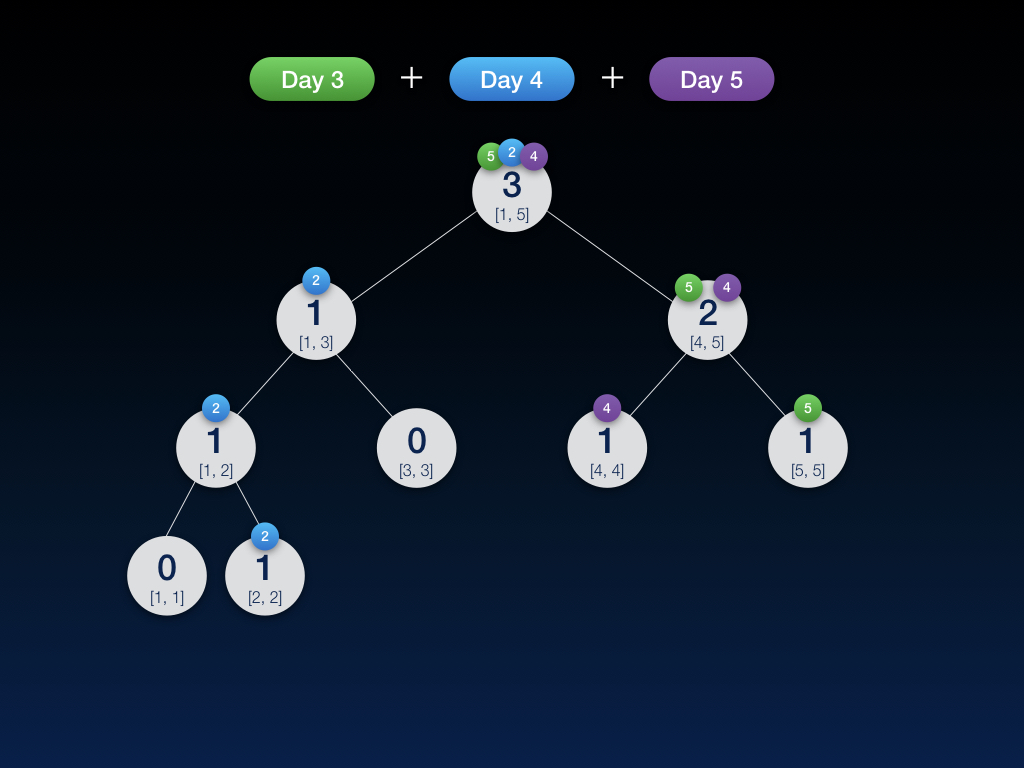### 主席树实战：求解区间第 K 大

• 若 K 小于等于左子树上的数值 sum，则递归到左子树找第 K 小
• 否则递归到右子树找第 K-sum 小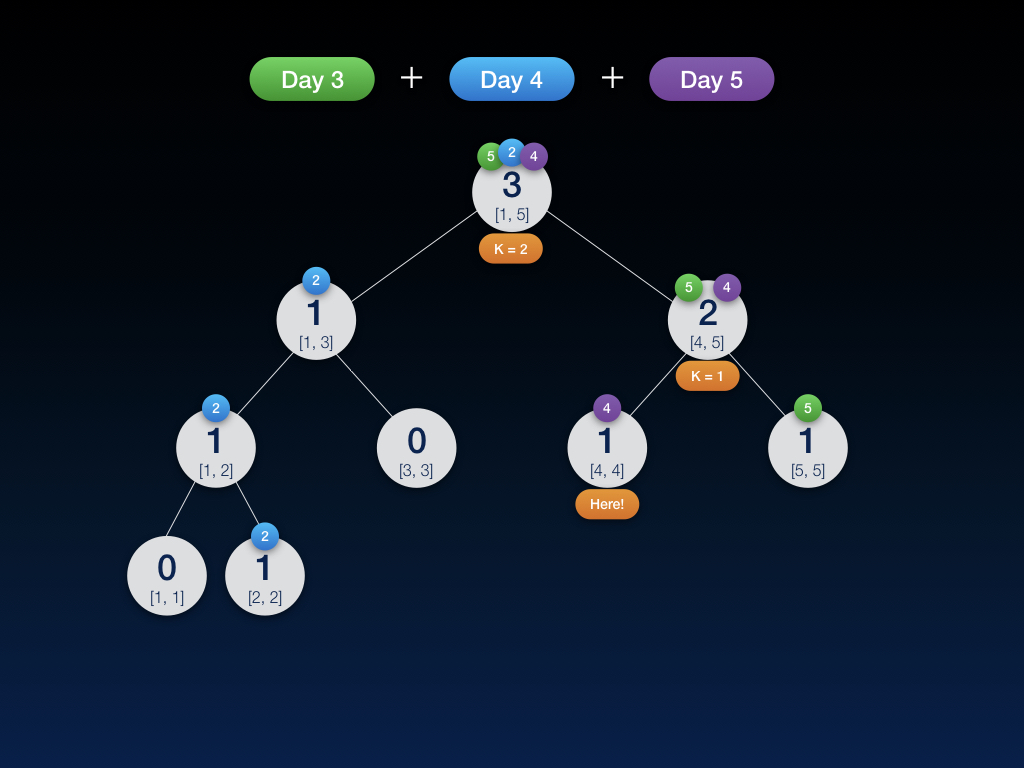WTF?

• A：控制不当
• B：玄学
• C：RP 问题
• D：无可奉告

• E：爆了内存 ✔️

### Code

#include <cstdio>
#include <algorithm>
using namespace std;

const int MAXN = 100001;

// 本代码中所有数据均按从下标 1 开始存放

// 主席树中的线段树结点，sum 表示此区间内元素个数
struct node {
int sum, l, r;
} hjt[MAXN*40];

int a[MAXN], sorted[MAXN], num;    // sorted: 离散化后的数组 num: 离散化后的数组长度
int root[MAXN], cnt;    // root: 主席树中用来保存每棵线段树树根的数组 cnt: 线段树结点数（用于数组方式动态开点）

// 查找离散化之后的下标
int GetIdx(int v) {
return lower_bound(sorted+1, sorted+1+num, v) - sorted;
}

// 初始化
void Init() {
cnt = 0;
}

// 创建结点
inline int CreateNode(int sum, int l, int r) {
int idx = ++cnt;
hjt[idx].sum = sum;
hjt[idx].l = l;
hjt[idx].r = r;

return idx;
}

// 新建一棵线段树，只沿更新路径新建出较上个版本有修改的结点
// 调用参数
// root: 插入后新生成的线段树的根结点会赋值到 root 中存储
// pre_rt: 上一棵线段树的根
// pos: 本次要插入的数在线段树中的位置
// l, r: 递归参数。默认填写 1, num
void Insert(int &root, int pre_rt, int pos, int l, int r) {
// 动态创建结点，直接根据上一个版本复制对应的结点，sum+1
root = CreateNode(hjt[pre_rt].sum+1, hjt[pre_rt].l, hjt[pre_rt].r);
if(l == r) return;
int m = (l+r) >> 1;
if(pos <= m)
Insert(hjt[root].l, hjt[pre_rt].l, pos, l, m);
else Insert(hjt[root].r, hjt[pre_rt].r, pos, m+1, r);
}

// 本函数适用于查询区间 [l, r] 中的第 k 小。通常需要自行变通
// 调用参数
// s, e: 要查询区间所需的两个线段树的根，如要查询区间 [l, r]，则传入 root[l-1], root[r]
// k: 要查询区间第几小
// l, r: 递归参数。默认填写 1, num
int Query(int s, int e, int k, int l, int r) {
if(l == r) return l;
int m = (l+r) >> 1;
int sum = hjt[hjt[e].l].sum - hjt[hjt[s].l].sum;    // 计算左子树的元素数量
if(k <= sum)    // 如果 k <= sum，则 k 在左子树，否则在右子树
return Query(hjt[s].l, hjt[e].l, k, l, m);
else return Query(hjt[s].r, hjt[e].r, k-sum, m+1, r);
}

int main(int argc, char const *argv[]) {
int n, m, l, r, k;
while(~ scanf("%d %d", &n, &m)) {
Init();
for(int i=1; i<=n; ++i) {
scanf("%d", &a[i]);
sorted[i] = a[i];
}
sort(sorted+1, sorted+1+n);    // 按值排序
num = unique(sorted+1, sorted+1+n) - (sorted+1);    // 去重，返回去重后的元素数量
for(int i=1; i<=n; ++i) {    // 按顺序插入，建立 n 棵权值线段树
Insert(root[i], root[i-1], GetIdx(a[i]), 1, num);
}
while(m--) {
scanf("%d %d %d", &l, &r, &k);
printf("%d\n", sorted[Query(root[l-1], root[r], k, 1, num)]);
}
}

return 0;
}

### 总结

• 基础结构：线段树
• 可持久化：每次插入保存一个新版本，利用共用数据减少时空消耗
• 动态开点，节省内存
• 时空复杂度 $O(nlogn)$

### 推荐练习题

• POJ 2104：区间第 K 大
• SPOJ DQUERY：区间不同数的个数
• SPOJ COT：树上路径第 K 大
• ZOJ 2112：区间第 K 大（带单点修改）

### 最后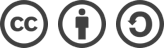bLue 创作，采用 知识共享署名 3.0，可自由转载、引用，但需署名作者且注明文章出处。